# KSEEB SSLC Class 10 Maths Solutions Chapter 3 Pair of Linear Equations in Two Variables Ex 3.2

n this chapter, we provide KSEEB SSLC Class 10 Maths Solutions Chapter 3 Pair of Linear Equations in Two Variables Ex 3.2 for English medium students, Which will very helpful for every student in their exams. Students can download the latest KSEEB SSLC Class 10 Maths Solutions Chapter 3 Pair of Linear Equations in Two Variables Ex 3.2 pdf, free KSEEB SSLC Class 10 Maths Solutions Chapter 3 Pair of Linear Equations in Two Variables Ex 3.2 book pdf download. Now you will get step by step solution to each question.

### Karnataka State Syllabus Class 10 Maths Chapter 3 Pair of Linear Equations in Two Variables Ex 3.2

Pair Of Linear Equations In Two Variables Class 10 Notes Exercise 3.2 Question 1.
Form the pair of linear equations in the following problems, and find their solutions graphically.
(i) 10 students of Class X took part in a Mathematics quiz. If the number of girls is 4 more than the number of boys, find the number of boys and girls who took part in the quiz.
(ii) 5 pencils and 7 pens together cost Rs. 50, whereas 7 pencils and 5 pens together cost Rs. 46. Find the cost of one pencil and that of one pen.
Solution:
(i) Strength of the X Std. is 10
Number of boys be ‘y’, then
number of girls be ‘x’ .
x + y = 10 …….. (i)
x = y + 4
∴ x – y = 4 ………. (ii)
From equations (i) + (ii)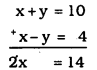x=142=7
Substituting the value of ‘x’ in eqn. (i),
x + y = 10
7 + y = 10
y = 10 – 7
y = 4.
∴ Number of girls, x = 7
Number of boys, y = 4.

(ii) Cost of each pencil be Rs. ‘x’
Cost of pen be Rs. ‘y’
5x + 7y = 50 ………. (i)
7x + 5y = 46 ……….. (ii)
From equation (i) + equation (ii)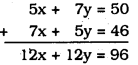∴ x + y = 8 …………… (iii)
from Eqn. (ii) – Eqn. (i),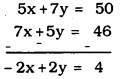∴ -x + y = 2 …………. (iv)
Eqn. (iii) + Eqn. (iv)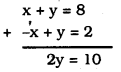∴ y = 5
Substituting the value of ‘y’ in eqn. (i)
x + y = 8
x + 5 = 8
∴ x = 8 – 5 x = 3
∴Cost of each pencil is Rs. 3.
Cost of each pen is Rs. 5.

KSEEB Solutions For Class 10 Maths Pair Of Linear Equations In Two Variables Question 2.
On comparing the ratios a1a2,b1b2 and c1c2 find out whether the lines representing the following pairs of linear equations intersect at a point, are parallel or coincident:
i) 5x – 4y + 8 = 0
7x + 6y – 9 = 0
ii) 9x + 3y + 12 = 0
18x + 6y + 24 = 0
iii) 6x – 3y + 10 = 0
2x – y + 9 = 0
Solution:
i) 5x – 4y + 8 = 0 a1= 5, b1 = -4, C1= 8
7x + 6y – 9 = 0 a2 = 7, b2= 6, c2= -9
a1a2=57b1b2=−46c1c2=8−9
Here, a1a2≠b1b2
∴ Lines representing the pairs of linear equations intersect at a point.

ii) 9x + 3y + 12 = 0
18x + 6y + 24 = 0
a1 =9, b1 = 3, c1 = 12
a2 = 18, b2 = 6, c2 = 24
a1a2=918=12b1b2=36=12
c1c2=1224=12
Here, a1a2=b1b2=c1c2
∴ Representation of lines graphically are coincident.

iii) 6x – 3y + 10 = 0
2x – y + 9 = 0
Here, a1 = 6, b1 = -3, c1 = 10
a2 = 2, b2 = -1, c2 = 9
a1a2=62=31b1b2=−3−1=31
c1c2=109
Here, a1a2=b1b2≠c1c2
∴ Representation of lines graphically is parallel.

KSEEB Solutions For Class 10 Maths Question 3.
On compairing the ratios a1a2,b1b2 and c1c2 find out whether the following pair of linear equations are consistent, or inconsistent.
i) 3x + 2y = 5; 2x – 3y = 7
ii) 2x – 3y = 8; 4x-6y = 9
iii) 32x+53y=7:9x−10y=14
iv) 5x – 3y = 11: -10x – 6y = -22
v) 43x+2y=8;2x+3y=12
Solution:
i) 3x + 2y = 5 ⇒ 3x + 2y – 5 = 0
2x – 3y = 7 ⇒ 2x – 3y – 7 = 0
Here, a1 = 3, b1 = 2, c1 = -5
a2 = 2, b2 = -3, c2 = -7
a1a2=32b1b2=−23c1c2=−5−7=57
Here, a1a2≠b1b2
∴ Graphical representation is intersecting lines.
∴ Pair of linear equations are consistent.

ii) 2x – 3y = 8 ⇒ 2x – 3y – 8 = 0
4x – 6y = 9 ⇒ 4x – 6y – 9 = 0
a1 = 2, b1 = -3, c1 = -8
a2 = 4, b2 = -6, c2 = -9
a1a2=24=12b1b2=−3−6=12
c1c2=−8−9=89
Here, a1a2=b1b2≠c1c2
∴ Graphical representation is parallel lines.
∴ Equations are inconsistent.

iii) 32x+53y=732x+53y−7=0
9x – 10y = 14 ⇒ 9x – 10y – 14 = 0
a1=32,b1=53,c1=−7
a2 = 9, b2 = -10, c2 = -17
a1a2=32×19b1b2=53×−16
c1c2=−7−14=714=12
Here, a1a2≠b1b2
∴ Pair of equations are consistent

iv) 5x – 3y = 11 ⇒ 5x – 3y – 11 = 0
-10x + 6y = – 22 ⇒ -10x + 6y + 22 = 0
a1 = 5, b1 = -3, c1 = -11
a2 = -10, b2 = 6, c2 = -22
a1a2=5−10=−12b1b2=−36=−12
c1c2=−1122=−12
Here, a1a2=b1b2=c1c2
∴ Pair of equations are consistent
∴ Graphical representation is coninciding.

v) 43x+2y=843x+26−8=0
2x + 3y = 12 ⇒ 2x + 3y – 12 = 0
a1=43,b1=2,c1=−8
a2 = 2, b2 = 3, c2 = -12
a1a2=43×12=16b1b2=23
c1c2=−8−12=23
Here, a1a2≠b1b2
∴ Pair of equations are consistent

Pair Of Linear Equations Exercise 3.2 KSEEB Solutions Question 4.
Which of the following pairs of linear equations are consistent/inconsistent? If consistent obtain the solution graphically
(i) x + y = 5, 2x + 2y = 10
(ii) x-y = 8 3x-3y= 16
(iii) 2x + y – 6 = 0 4x – 2y – 4 = 0
(iv) 2x – 2y – 2 = 0 4x – 3y – 5 = 0
Solution:
(i) x + y = 5 ⇒ x + y – 5 = 0
2x + 2y = 10 ⇒ 2x + 2y – 10 = 0
a1 = 1, b1 = 1, c1 = -5
a2 = 2, b2 = 2, c2 = -10
a1a2=12b1b2=12c1c2=−5−10=12
Here, a1a2=b1b2=c1c2
∴ Pair of equations are consistent
(i) x + y = 5
y = 5 – x

(ii) 2x + 2y = 10
x + y = 5
y = 5 – x

∴ We can give any value for ‘x’, i.e., solutions are infinite.
∴ Solution, P (5, 0) x = 5, y = 0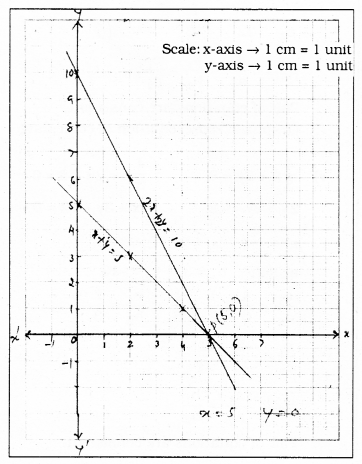(ii) x – y = 8 ⇒ x – y – 8 = 0
3x – 3y = 16 ⇒ 3x – 3y – 16 = 0
Here, a1a2=13b1b2=−1−3=13
c1c2=−8−16=12
a1a2=b1b2≠c1c2
∴ Linear equations are inconsistenttent.
∴ Algebraically it has no solution.
Graphical representation → Parallel Lines.
(i) x – y = 8
-y = 8 – x
y = -8 + x

(ii) 3x – 3y = 16
-3y = 16 – 3x
3y = -16 + 3x
y=−16+3×3

No solution because it is inconsistent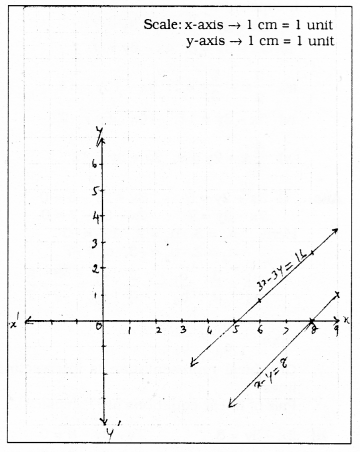(iii) 2x + y – 6 = 0
4x – 2y – 4 = 0
Here a1 = 2, b1 = 1, c1 = -6
a2 = 4, b2 = -2, c2 = -4
a1a2=24=12b1b2=1−2
c1c2=−6−4=32
Here, a1a2≠b1b2
Pair of equations are consistent. Algebraically both lines intersect.
Graphical Representation :
(i) 2x + y = 6
y = 6 – 2x

(ii) 4x – 2y – 4 = 0
4x – 2y = 4
-2y = 4 – 4x
2y = -4 + 4x
y=−4+4×2

Solution: intersecting point, P (2, 2) i.e., x = 2, y = 2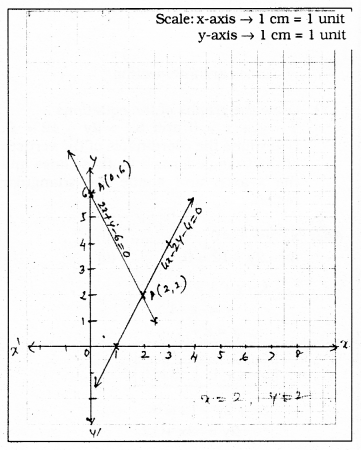(iv) 2x – 2y – 2 = 0
4x – 3y – 5 = 0
a1 = 2, b1 = -2, c1 = -2
a2 = 4, b2 = -3, c2 = -5
a1a2=24=12b1b2=−2−3=23
c1c2=−2−5=25
Here, a1a2≠b1b2
Pair of equations are consistent.
∴ Algebraically both lines intersect.
Graphical Representation :
(i) 2x – 2y – 2 =0
2x – 2y = 2
-2y = 2 – 2x
2y = -2 + 2x
y=−2+2×2
∴ y = – 1 + x

(ii) 4x – 3y – 5 = 0
4x – 3y = 5
-3y = 5 – 4x
3y = -5 + 4x
y=−5+4×3

Solution: P(2, 1) i.e., x = 2, y = 1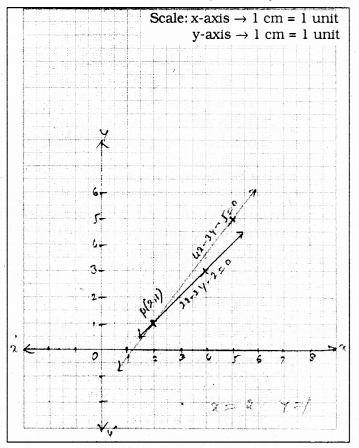Pair Of Linear Equations In Two Variables KSEEB Solutions Question 5.
Half the perimeter of a rectangular garden, whose length is 4m more than its width, is 36m. Find the dimensions of the garden.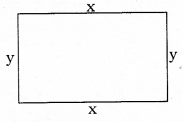Solution:
Length of rectangular garden be ‘x’ m.
Breadth of rectangular garden be ‘y’ m, then
Length of the garden is 4m more than its width.
x = y + 4 ……….. (i)
x – y = 4
Half of the circumference is 36 m.
2x+2y2=36
2x + 2y = 72
x + y = 36 …………… (ii)
∴ x – y = 4 (i)
x + y = 36 (ii)
From eqn. (i) + eqn. (ii)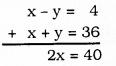x=402
∴ x = 20 m.
Substituting the value of ’x’ in eqn. (i)
x – y = 4
20 – y = 4
-y = 4 – 20
-y = -16
y = 16 m.
∴ Length of the garden = 20 m.
Breadth of the garden = 16 m.

Pair Of Linear Equations Exercise 3.2 Question 6.
Given the linear equation 2x + 3y – 8 = 0, write another linear equation in two variables such that the geometrical representation of the pair so formed is :
(i) intersecting lines
(ii) parallel lines
(iii) coincident lines.
Solution:
Linear equation is 2x + 3y – 8 = 0.
(i) Linear equations for intersecting lines:
2x + 3y – 8 = 0
3x + 2y – 7 = 0
a1 = 2, b1 = 3, c1 = -8
a= 3, b2 = 2, c2 = -7
a1a2=23b1b2=32
Here, when a1a2≠b1b2 Geometrical representation is intersecting lines.

(ii) For Parallel lines :
2x + 3y – 8 = 0
2x + 3y – 12 = 0
a1 = 2, b1 = 3, c1= -8
a1 = 2, b1 = 3, c1 = -12
a1a2=22=11b1b2=33=11
c1c2=−8−12=812=23
Here, a1a2=b1b2≠c1c2 hence
Graphical representation is parallel lines.

(iii) For Intersecting lines :
2x + 3y – 8 = 0
4x + 6y – 16 = 0
a1 = 2, b1 = 3, c1 = -8
a1 = 4, b1 = 6, c1 = -16
a1a2=24=12b1b2=36=12
c1c2=−8−16=12
Here,
∴ Lines are intersecting.

10th Maths Pair Of Linear Equations In Two Variables Exercise 3.2 Question 7.
Draw the graphs of the equations x – y + 1 =0 and 3x + 2y – 12 = 0. Determine the coordinates of the vertices of the triangle formed by these lines and the x-axis, and shade the triangular region.
Solution:
x – y + 1 = 0 ………. (i)
3x – 2y – 12 = 0 ……….. (ii)
x – y + 1 = 0
-y = -x -1
y = x + 1

3x + 2y – 12 = 0
2y = -3x + 12
y=−3x+122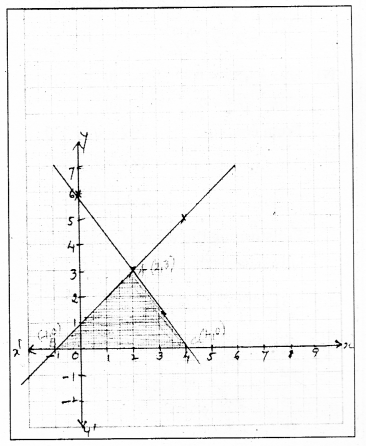Graphs of these two equations intersect at A. Vertices formed for ∆ABC are,
A (2, 3), B (-1, 0), C (4, 0).

All Chapter KSEEB Solutions For Class 10 Maths

—————————————————————————–

All Subject KSEEB Solutions For Class 10

*************************************************

I think you got complete solutions for this chapter. If You have any queries regarding this chapter, please comment on the below section our subject teacher will answer you. We tried our best to give complete solutions so you got good marks in your exam.

If these solutions have helped you, you can also share kseebsolutionsfor.com to your friends.

Best of Luck!!